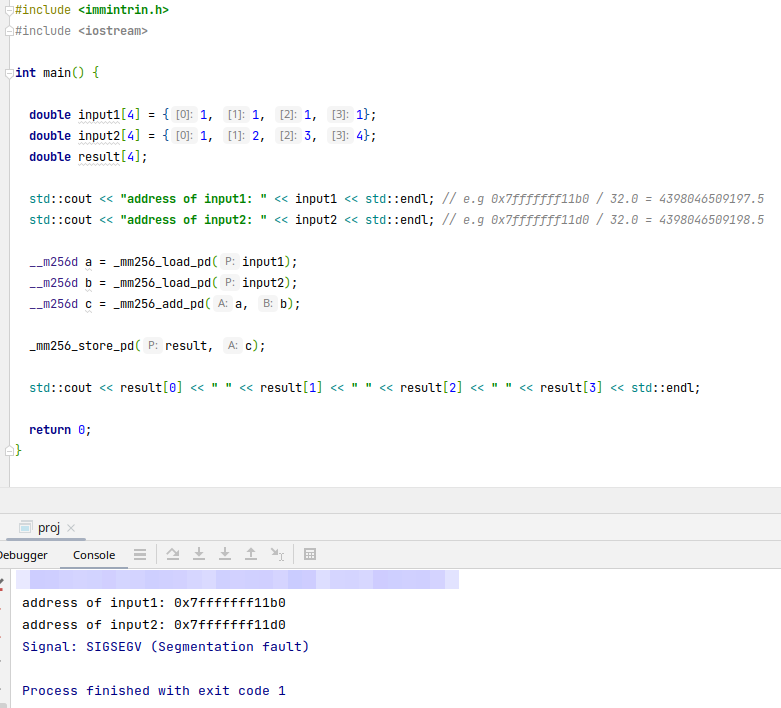## Alignment Issue

• 指令的操作数的地址没有对齐，则会报错（段错误），例如AVX指令集需要32位内存对齐（即该操作数的地址需要被32整除）• 指定变量的内存对齐

```// e.g. 指定分配的内存地址能被32整除
// 等价于： __attribute__ ((aligned (32))) double input1 = {1, 1, 1, 1};
alignas(64) double input1 = {1, 1, 1, 1};
```
• 查看当前CPU支持的指令集

```
span.prompt1:before {
content: "\$ ";
}
span.prompt2:before {
content: "# ";
}
cat /proc/cpuinfo
```

## GenericProgramming

• 支持多种类型的算法，比如`std::sort()`；基于容器的算法

• 标准库：`algorithm``numeric``ranges`

### Q&A

• C++中内建数据类型不支持方法

• 计算逻辑存在相似性，避免重复定义

## MetaProgramming

### 判断某个类型的属性

```#include <iostream>
#include <type_traits>
using namespace std;

int main() {
cout << boolalpha << endl;
cout << "int is copy-assignable? " << is_copy_assignable<int>::value << endl;
}
```

### 得到某个类型的大小

• `sizeof(type/object)`：用来获取`对象``类型所对应的对象`的大小（单位：字节）

• `sizeof`无法测动态数组的内存大小，因为得到的只是指向首元素的指针而不是数组名（数组名也是个地址，但其类型指向整个数组）"Used when actual size of the object must be known"

```auto ptr = new bool;
cout << sizeof(ptr) << endl; // 8
cout << sizeof(*ptr) << endl; // 1（获得指向首元素的对象，并得其大小）
bool ptr2;
cout << sizeof(ptr2) << endl; // 40 （sizeof不会触发数组类型到指针的类型转换）

// sizeof(vector)为24：对应的元素为三个指针（3×8字节）：_M_start, _M_finish, _M_end_of_storage
```

### 判断两个变量是否是相同类型/某个变量是否某个类型

```#include <type_traits>
using namespace std;

int main() {
vector<int> arr(5);
// 注意使用尖括号
cout << is_same_v<decltype(arr.size()), unsigned long> << endl;

// c11判断类型
int a = {1, 2, 3};
cout << typeid(a).name() << endl;
// c17判断类型是否相同（type_traits）
cout << is_same_v<decltype(a), int *> << endl;
}
```

## Q&A

### 为什么设置左闭右开区间作为规范？

• 在实践过程中发现左闭右开和左开右闭，都能够实现区间相减（右区间-左区间）而得到元素的个数

• 若要区间包含上自然数0时，左开右闭的话会让区间下界包含非自然数

### Off-By-One Error

• 要构建一个120块石头的时间金字塔，每隔十年放一块石头。共需要多少年完成？

共有120块石头，放了120次，间隔了119次，耗时1190年

• 一年级到六年级读完，要多少年？

[1, 7) 共跨越6个年级，每个年级需要读1年，共6年

• 元素（序号2）和元素（序号6）之间相差多少元素：(2, 6) 相差4-1=3个元素

• 区间和元素个数

`左开右开( )`

`r - l - 1`

`左闭右开[ )`

`r - l`

`左闭右闭[ ]`

`r - l + 1`